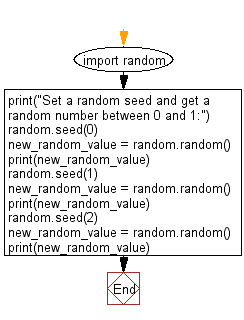﻿ Python: Set a random seed and get a random number between 0 and 1 - w3resource# Python: Set a random seed and get a random number between 0 and 1

## Python module: Exercise-9 with Solution

Write a Python program to set a random seed and get a random number between 0 and 1.

Sample Solution:

Python Code:

``````import random
print("Set a random seed and get a random number between 0 and 1:")
random.seed(0)
new_random_value = random.random()
print(new_random_value)
random.seed(1)
new_random_value = random.random()
print(new_random_value)
random.seed(2)
new_random_value = random.random()
print(new_random_value)
```
```

Sample Output:

```Set a random seed and get a random number between 0 and 1:
0.8444218515250481
0.13436424411240122
0.9560342718892494
```

Flowchart:## Visualize Python code execution:

The following tool visualize what the computer is doing step-by-step as it executes the said program:

Python Code Editor:

Have another way to solve this solution? Contribute your code (and comments) through Disqus.

What is the difficulty level of this exercise?

Test your Python skills with w3resource's quiz

﻿

## Python: Tips of the Day

For-else construct useful when searched for something and find it:

```# For example assume that I need to search through a list and process each item until a flag item is found and
# then stop processing. If the flag item is missing then an exception needs to be raised.

for i in mylist:
if i == theflag:
break
process(i)
else:
raise ValueError("List argument missing terminal flag.")
```